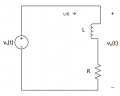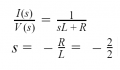# RL Transfer Function: Struggling with what to do with wave Input V

#### worthia2

Joined Dec 21, 2019
1
I have exams next month, our lecturer left a bit uncovered this section being one of them so any help is appreciated.
This is the circuit:The R = 2Ω and L = 2H. Vs(t) = 12cos(50t + 30°).
I had to find the transfer function that relates iL(t) to vS(t). I did this :I've looked through all her notes but she doesn't explain how to find and use the phase and magnitude of the "transfer function at frequency of input voltage" (second part of question).
Any guidance at all is great, just want to be able to understand how to solve this question and questions like them for the exam.
Thanks

#### MisterBill2

Joined Jan 23, 2018
9,854
First you need to find the impedance of that RL series combination. That is the sum of the resistance and the inductive reactance, for which you need to know the frequency. The impedance is the vector sum of those values.

#### Papabravo

Joined Feb 24, 2006
17,307
So do you know what the frequency of the cosine wave is?
Do you know how to convert a frequency (f) in Hertz (Hz) to angular frequency (ω) in radians/sec?

Your ability to solve such questions depends on knowing the answers to these two question without having to think about it.

Knowing Euler's identity is also helpful

$\huge e^{j\omega t}e^{\phi} =e^{j\omega t+\phi} = cos(\omega t + \phi) + jsin(\omega t +\phi)$

So your problem has the real part of a complex exponential.

Last edited:

#### MisterBill2

Joined Jan 23, 2018
9,854
So do you know what the frequency of the cosine wave is?
Do you know how to convert a frequency (f) in Hertz (Hz) to angular frequency (ω) in radians/sec?

Your ability to solve such questions depends on knowing the answers to these two question without having to think about it.

Knowing Euler's identity is also helpful

$\huge e^{j\omega t}e^{\phi} =e^{j\omega t+\phi} = cos(\omega t + \phi) + jsin(\omega t +\phi)$

So your problem has the real part of a complex exponential.
Yes, the solution has both an amplitude and a phase angle that is not zero. And I= E/Z, where Z=(2) +XL with XL= the inductive reactance of the 2H inductor at the frequency of the wave.